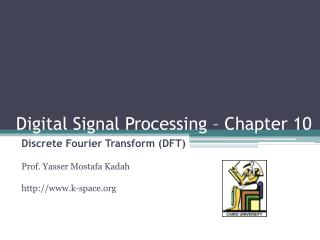# Digital Signal Processing – Chapter 10 - PowerPoint PPT PresentationDownload PresentationDigital Signal Processing – Chapter 10

Digital Signal Processing – Chapter 10
Download Presentation## Digital Signal Processing – Chapter 10

- - - - - - - - - - - - - - - - - - - - - - - - - - - E N D - - - - - - - - - - - - - - - - - - - - - - - - - - -
##### Presentation Transcript

1. Digital Signal Processing – Chapter 10 Discrete Fourier Transform (DFT) Prof. Yasser MostafaKadah http://www.k-space.org

2. Textbook Luis Chapparo, Signals and Systems Using Matlab, Academic Press, 2011.

3. Discrete-Time Fourier Transform • Sampled time domain signal has a Fourier transform that is periodic • We should take that into consideration in the definition of Fourier transform for discrete-time signals • Define Discrete-time Fourier Transform (DTFT) as: Forward DTFT Inverse DTFT

4. Notes on DTFT • DTFT is just one period of the continuous Fourier transform of the sampled signal • Z-transform is related to the DTFT as follows: • DTFT is the values on the unit circle in z-Transform • Unit circle must be included in the ROC • Problem: the sampled signal is still infinite in length • Impossible to obtain using computers • Consider cases when the signal has compact support

5. x(n) n Discrete Fourier Transform (DFT) • Periodic and sampled time domain signal has a Fourier transform that is both periodic and sampled • DFT is Defined as the Fourier transform of a signal that is both discrete and periodic • Fourier transform will also be discrete and periodic • Can assume periodicity if we have a finite duration of a signal: better than zero assumption since it allows reducing the frequency domain to a sampled function

6. DFT Formula • Given a periodic signal x[n] of period N, its DFT is given by, • Its inverse is given by, • Both X[k] and x[n] are periodic of the same period N.

7. Computation of DFT Using FFT • A very efficient computation of the DFT is done by means of the FFT algorithm, which takes advantage of some special characteristics of the DFT • DFT requires O(N2) computations • FFT requires O(N log2N) computations • Both compute the same thing • It should be understood that the FFT is not another transformation but an algorithm to efficiently compute DFTs. • Always remember that the signal and its DFT are both periodic

8. Improving Resolution in DFT • When the signal x[n] is periodic of period N, the DFT values are normalized Fourier series coefficients of x[n] that only exist for the N harmonic frequencies {2k/N}, as no frequency components exist for any other frequencies • The number of possible frequencies depend on the length L chosen to compute its DFT. • Frequencies at which we compute the DFT can be seen as frequencies around the unit circle in the z-plane • We can improve the frequency resolution of its DFT by increasing the number of samples in the signal without distorting the signal by padding the signal with zeros • Increase samples of the units circle

9. Zero-Padding Example Matlab Code N= 32; N2= 128; n=0:N-1; n2= 0:N2-1; x= zeros(N,1); x(1:16)= 1; X= fftshift(fft(x)); X2= fftshift(fft(x,N2)); subplot(3,1,1) stem(n,x) subplot(3,1,2) stem(n,abs(X)) subplot(3,1,3) stem(n2,abs(X2)) Original Signal No zero-padding 32-Point FFT With zero-padding 128-Point FFT

10. Phase in DFT • Output of DFT is a set of complex numbers • Phase is just as important as magnitude in DFT • Time shift amounts to linear phase • Phase wrapping occurs for large shifts • Linear phase can be unwrapped to its correct form using the “unwrap” function of Matlab N= 32; x= zeros(N,1); x(11:15)= [0.2 0.5 1 0.5 0.2]; X= fftshift(fft(x)); subplot(4,1,1); stem(1:N,x); title('Signal') subplot(4,1,2); plot(abs(X)); title('DFT Magnitude') subplot(4,1,3); plot(angle(X)); title('Computed Phase') subplot(4,1,4); plot(unwrap(angle(X))); title('Unwrapped Phase')

11. Phase in DFT: Examples More Time Shift Less Time Shift Steeper linear phase Slower linear phase

12. Linear vs. Circular Convolution • Convolution in continuous and discrete time aperiodic signals is called linear convolution • Convolution can be calculated as a multiplication in the frequency domain • Convolution property of the Fourier transform • Problem: when using DFT to compute convolution of aperiodic signals, the periodicity assumption results in errors in computing the convolution • Signal outside the N points used is not zero: periodic extension of the N points disturb the computation • What we compute is called circular convolution

13. Linear vs. Circular Convolution • Given x[n] and h[n] of lengths M and K, the linear convolution sum y[n] of length N=(M+K-1) can be found by following these three steps: • Compute DFTs X[k] and H[k] of length LN for x[n] and h[n] using zero-padding • Multiply them to get Y[k]= X[k]H[k]. • Find the inverse DFT of Y[k] of length L to obtain y[n]

14. Linear vs. Circular Convolution: Example N1= 16; N2= 16; N= 32; % N>N1+N2-1 x1= zeros(N1,1); x2= zeros(N2,1); x1(1:4)= 1; x2(:)= 1; X1= fft(x1,N1); X2= fft(x2,N1); X_circ= X1.*X2; x_circ= ifft(X_circ); X1= fft(x1,N); X2= fft(x2,N); X_lin= X1.*X2; x_lin= ifft(X_lin); figure(1); subplot(4,1,1); stem(1:N1,x1) subplot(4,1,2); stem(1:N2,x2) subplot(4,1,3); stem(1:N1, abs(x_circ)) title('Circular Convolution'); subplot(4,1,4); stem(1:N,abs(x_lin)) title('Circular Convolution');

15. Fourier Transformations Chart Most General Form: others are special cases Only one that can be done on a computer

16. Problem Assignments • Problems: 10.12, 10.26, 10.27, 10.29 • Partial Solutions available from the student section of the textbook web site